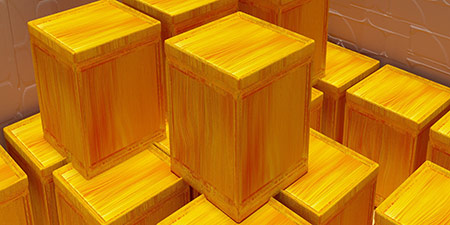# US Cup (US.cup) to Milliliter (ml)

Volume ﹣ Converter US Cup to Milliliter

Here you can convert the Volume unit US Cup into the unit Milliliter and vice versa you can convert Milliliter into US Cup. By clicking the "Swap units" icon, you will always obtain the desired conversion in the calculation result, i.e., US.cup to ml or ml to US.cup. With the following calculator you can also calculate any other Volume unit.

The US Cup is still frequently used in American cooking recipes. It is equivalent to about 237 milliliters and differs from the UK cup, which is equivalent to about 284 milliliters.

16 US Cup = 8 US Pint = 4 US Quart = 1 US Gallon

US Cup and also the British Cup are fixed units of measurement, unlike e.g. in German cookbooks, where the literal translation of "Cup", i.e., cup is only an approximate unit of measurement. This should be taken into account when translating recipes, for example.

One milliliter (ml; spelled millilitre in Commonwealth English and milliliter in American English) corresponds to one cubic centimeter (cm³) and thus to the volume of a cube with an edge length of 1 cm.

The milliliter is not part of the International System of Units (SI), but is approved for use with the SI because it is a fraction of the approved liter.

## Basis for conversion US Cup (US.cup) to Milliliter (ml) and vice versa

The abbreviation for the "Volume unit US Cup" is US.cup. The abbreviation for the "Volume unit Milliliter" is ml.

## Formula for the conversion of US Cup (US.cup) to Milliliter (ml) and vice versa

The calculation from US Cup to Milliliter shall be made using the following conversion formula:

Conversion formula US Cup to Milliliter

Determine the number of Milliliter from US Cup

US Cup × 236.5882365

## Formula for the conversion of Milliliter (ml) to US Cup (US.cup)

The calculation from Milliliter to US Cup shall be made using the following conversion formula:

Conversion formula Milliliter to US Cup

Determine the number of US Cup from Milliliter

Milliliter × 0.0042267528377304

## Overview table : How many US Cup are in a Milliliter ?

US Cup US.cup ⇒ Milliliter ml
0.01 US.cup  are  2.36588 ml
0.02 US.cup  are  4.73176 ml
0.03 US.cup  are  7.09764 ml
0.04 US.cup  are  9.46352 ml
0.05 US.cup  are  11.82941 ml
0.06 US.cup  are  14.19529 ml
0.07 US.cup  are  16.56117 ml
0.08 US.cup  are  18.92705 ml
0.09 US.cup  are  21.29294 ml
0.10 US.cup  are  23.65882 ml
0.20 US.cup  are  47.31764 ml
0.30 US.cup  are  70.97647 ml
0.40 US.cup  are  94.63529 ml
0.50 US.cup  are  118.29411 ml
0.60 US.cup  are  141.95294 ml
0.70 US.cup  are  165.61176 ml
0.80 US.cup  are  189.27058 ml
0.90 US.cup  are  212.92941 ml
1 US.cup  corresponds to  236.58823 ml
2 US.cup  are  473.17647 ml
3 US.cup  are  709.76470 ml
4 US.cup  are  946.35294 ml
5 US.cup  are  1 182.94118 ml
6 US.cup  are  1 419.52941 ml
7 US.cup  are  1 656.11765 ml
8 US.cup  are  1 892.70589 ml
9 US.cup  are  2 129.29412 ml
10 US.cup  are  2 365.88236 ml
20 US.cup  are  4 731.76473 ml
30 US.cup  are  7 097.64709 ml
40 US.cup  are  9 463.52946 ml
50 US.cup  are  11 829.41182 ml
60 US.cup  are  14 195.29419 ml
70 US.cup  are  16 561.17655 ml
80 US.cup  are  18 927.05892 ml
90 US.cup  are  21 292.94128 ml
100 US.cup  are  23 658.82365 ml
200 US.cup  are  47 317.64730 ml
300 US.cup  are  70 976.47095 ml
400 US.cup  are  94 635.29460 ml
500 US.cup  are  118 294.11825 ml
600 US.cup  are  141 952.94190 ml
700 US.cup  are  165 611.76555 ml
800 US.cup  are  189 270.58920 ml
900 US.cup  are  212 929.41285 ml
1 000 US.cup  are  236 588.23650 ml

## Overview table : How many Milliliter are in a US Cup ?

Milliliter ml ⇒ US Cup US.cup
0.01 ml  are  0.00004 US.cup
0.02 ml  are  0.00008 US.cup
0.03 ml  are  0.00012 US.cup
0.04 ml  are  0.00016 US.cup
0.05 ml  are  0.00021 US.cup
0.06 ml  are  0.00025 US.cup
0.07 ml  are  0.00029 US.cup
0.08 ml  are  0.00033 US.cup
0.09 ml  are  0.00038 US.cup
0.10 ml  are  0.00042 US.cup
0.20 ml  are  0.00084 US.cup
0.30 ml  are  0.00126 US.cup
0.40 ml  are  0.00169 US.cup
0.50 ml  are  0.00211 US.cup
0.60 ml  are  0.00253 US.cup
0.70 ml  are  0.00295 US.cup
0.80 ml  are  0.00338 US.cup
0.90 ml  are  0.00380 US.cup
1 ml  corresponds to  0.00422 US.cup
2 ml  are  0.00845 US.cup
3 ml  are  0.01268 US.cup
4 ml  are  0.01690 US.cup
5 ml  are  0.02113 US.cup
6 ml  are  0.02536 US.cup
7 ml  are  0.02958 US.cup
8 ml  are  0.03381 US.cup
9 ml  are  0.03804 US.cup
10 ml  are  0.04226 US.cup
20 ml  are  0.08453 US.cup
30 ml  are  0.12680 US.cup
40 ml  are  0.16907 US.cup
50 ml  are  0.21133 US.cup
60 ml  are  0.25360 US.cup
70 ml  are  0.29587 US.cup
80 ml  are  0.33814 US.cup
90 ml  are  0.38040 US.cup
100 ml  are  0.42267 US.cup
200 ml  are  0.84535 US.cup
300 ml  are  1.26802 US.cup
400 ml  are  1.69070 US.cup
500 ml  are  2.11337 US.cup
600 ml  are  2.53605 US.cup
700 ml  are  2.95872 US.cup
800 ml  are  3.38140 US.cup
900 ml  are  3.80407 US.cup
1 000 ml  are  4.22675 US.cup

## Source information

As source for the information in the 'Volume' category, we have used in particular:

## Last update

This page of the 'Volume' category was last edited or reviewed by Stefan Banse on February 20, 2023. It corresponds to the current status.

### Changes in this category "Volume"

• Publication of the topic Convert Volumes together with the corresponding texts.
• Extension of the Volume Converter by further units: Liquid ounce, cup, tablespoon and teaspoon